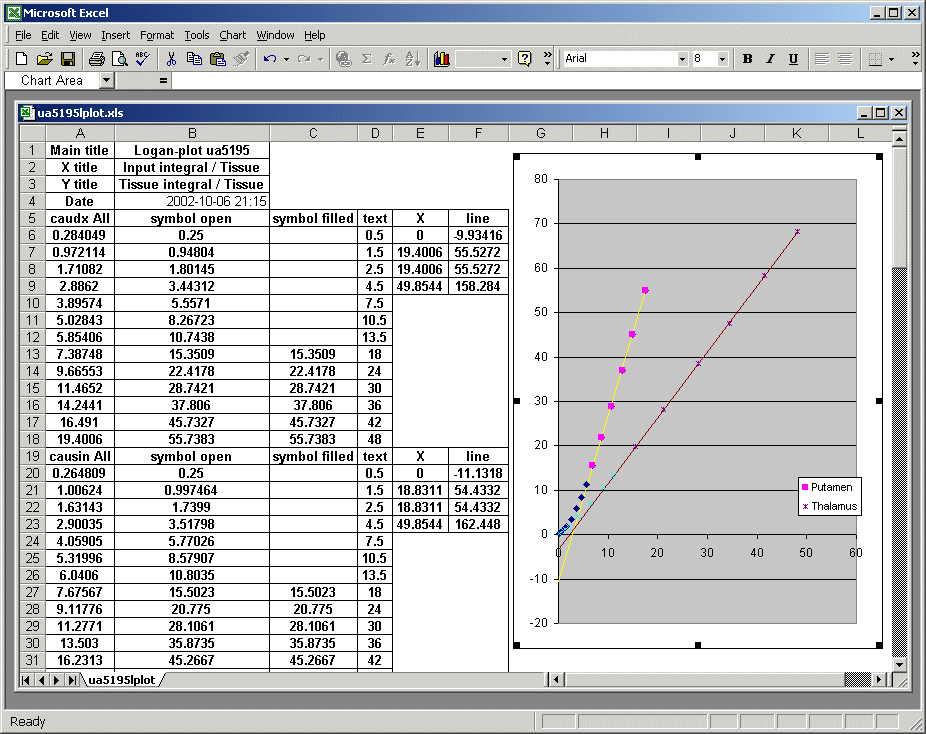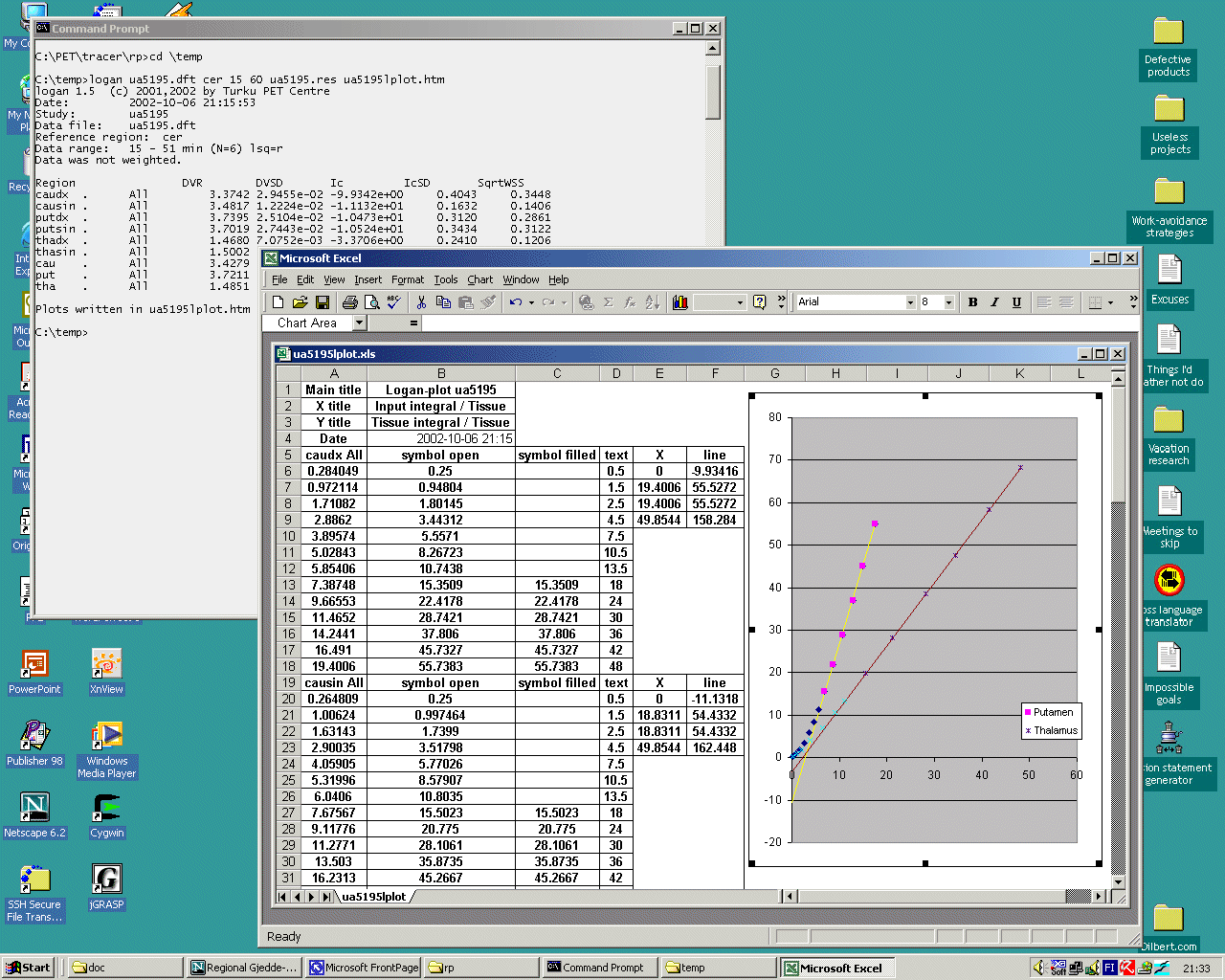# Drawing Patlak and Logan plots

When using multiple-time graphical analysis (MTGA) methods (Patlak, Logan, or Yokoi plots), the quality of linear fit, and that selected fit range is appropriate, must be checked visually in each study.

Carimas shows the Patlak and Logan plots automatically, when these models are applied to the regional data.

### Drawing plots in SVG format

Command-line programs patlak and logan can optionally save the plots in Scalable Vector Graphics files.

### Drawing plots in spreadsheet software

The plot data can optionally be saved in HTML table files when using patlak, logan, yokoi, or mpatlak. These *.htm or *.html files can then be imported for example in MS Excel, where the plot data and fitted lines can be drawn, or copied to Origin to make publication quality figures.

Plot data files contain several tables: First there is a table containing information for all plots: e.g. the axis titles, and the calculation date. Then there are the regional plots each in its own table. First column contains the x-axis plot values, the second contains all y-axis values, and the 3rd column contains only those y-column values that were used to fit the line. After that, there is a column containing PET frame mid times; this is useful in determining the time range when the plot is linear.

Next two columns, containing less rows, contain data that can be used to draw the fitted line. With the first two lines, a line to the end of fitted range can be drawn. Third row contains line coordinates to the end of all regional plot data (this is the same as the 2nd one, if line was fitted to the end of data). The 4th row can be used to extend the lines to same range for all regions.Screen captures of a Logan plot analysis of a raclopride study, when data is plotted in Excel.